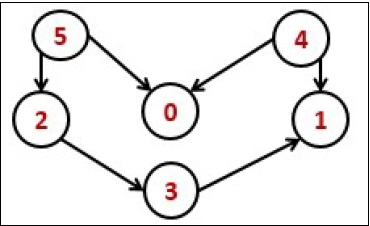Topological Sorting

AlgorithmsData StructureGraph Algorithms

The topological sorting for a directed acyclic graph is the linear ordering of vertices. For every edge U-V of a directed graph, the vertex u will come before vertex v in the ordering.As we know that the source vertex will come after the destination vertex, so we need to use a stack to store previous elements. After completing all nodes, we can simply display them from the stack.

Input and Output

Input:
0 0 0 0 0 0
0 0 0 0 0 0
0 0 0 1 0 0
0 1 0 0 0 0
1 1 0 0 0 0
1 0 1 0 0 0

Output:
Nodes after topological sorted order: 5 4 2 3 1 0

Algorithm

topoSort(u, visited, stack)

Input − The start vertex u, An array to keep track of which node is visited or not. A stack to store nodes.
Output − Sorting the vertices in topological sequence in the stack.

Begin
mark u as visited
for all vertices v which is adjacent with u, do
if v is not visited, then
topoSort(c, visited, stack)
done

push u into a stack
End

performTopologicalSorting(Graph)

Input − The given directed acyclic graph.
Output − Sequence of nodes.

Begin
initially mark all nodes as unvisited
for all nodes v of the graph, do
if v is not visited, then
topoSort(i, visited, stack)
done
pop and print all elements from the stack
End.

Example

#include<iostream>
#include<stack>
#define NODE 6
using namespace std;

int graph[NODE][NODE] = {
{0, 0, 0, 0, 0, 0},
{0, 0, 0, 0, 0, 0},
{0, 0, 0, 1, 0, 0},
{0, 1, 0, 0, 0, 0},
{1, 1, 0, 0, 0, 0},
{1, 0, 1, 0, 0, 0}
};

void topoSort(int u, bool visited[], stack<int>&stk) {
visited[u] = true;                //set as the node v is visited

for(int v = 0; v<NODE; v++) {
if(graph[u][v]) {             //for allvertices v adjacent to u
if(!visited[v])
topoSort(v, visited, stk);
}
}
stk.push(u);     //push starting vertex into the stack
}

void performTopologicalSort() {
stack<int> stk;
bool vis[NODE];

for(int i = 0; i<NODE; i++)
vis[i] = false;     //initially all nodes are unvisited

for(int i = 0; i<NODE; i++)
if(!vis[i])     //when node is not visited
topoSort(i, vis, stk);

while(!stk.empty()) {
cout << stk.top() << " ";
stk.pop();
}
}

main() {
cout << "Nodes after topological sorted order: ";
performTopologicalSort();
}

Output

Nodes after topological sorted order: 5 4 2 3 1 0